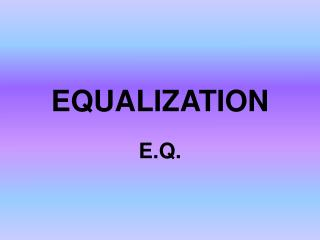Download PresentationEQUALIZATION

# EQUALIZATION

Download Presentation## EQUALIZATION

- - - - - - - - - - - - - - - - - - - - - - - - - - - E N D - - - - - - - - - - - - - - - - - - - - - - - - - - -
##### Presentation Transcript

1. EQUALIZATION E.Q.

2. What is equalization? • The manipulation of tone by increasing or decreasing frequency ranges with tone controls, filters or equalizers.

3. EQ has two main purposes: • to eliminate (cut) the part of a tone that we don’t want 2) to enhance (boost) the part of the tone that we do want

4. SOUND To understand how EQ works we must first understand how sound works.

5. Sound has three main components: 1) Amplitude (volume) 2) Frequency (fundamental pitch) 3) Timbre (overtone structure)

6. Amplitude • Amplitude expresses the amount of energy in a waveform • It is indicated by the height of the crest and depth of the trough in a waveform.

7. Frequency • Frequency is the number of times the wave completes its 360° cycle in one second. • Frequency is usually expressed as hertz (Hz). Units of a thousand Hz or higher are often stated as Kilohertz (kHz). example: 12 000 Hz = 12 kHz (or 12 k) • Human beings (at best) can hear frequencies that fall between 20 Hz and 20 000 Hz.

8. Timbre Timbre (tone colour) comes from the relative strength of overtones or partialsabove the fundamental pitch of a note.

9. Harmonic partials or overtones that belong to the fundamental pitch of low "C":

10. Flat EQ When no equalization is added to a tone we call this "flat".

11. Frequency ranges can be grouped from high to low as listed below: a) Brilliance (Highs) b) Presence (Highs) c) Upper Midrange (Mids) d) Lower Midrange (Mids) e) Bass (Bass) f) Sub-Bass (Bass)

12. There are two main types of equalization devices: • Parametric EQ 2) Graphic EQ

13. Parametric EQ These devices allow you to choose a specific frequency, adjust the bandwidth (from narrow to wide) and choose the amount that the frequency band is either boosted or cut.

14. Graphic EQ These devices have a slider for each frequency region. They are most often used as outboard devices to adjust the equalization of the entire mix all at once. A graphic EQ usually has 10, 15 or 31 sliders. A 10 slider EQ has one slider for each octave.